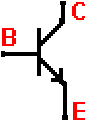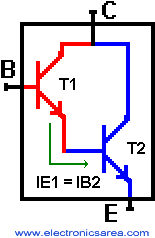# Darlington transistor

The Darlington transistor is a special type of transistor which has a high current gain. It consists of two internally bipolar transistors being connected in cascade.The T1 transistor delivers on its emitter terminal, a current that goes to the base of the T2 transistor. The current gain equation of a typical transistor is:  IE = ßxIB (collector current is equal to beta times the base current).

The picture shows a common transistor with its pin configuration. Analysing the picture:

• On the first transistor: IE1 = ß1 x IB1 (1),
• On the second transistor is: IE2 = ß2 x IB2 (2)From the picture, we can see that the emitter current of the T1 transistor is the same as the base current of the T2 transistor. Then IE1 = IB2 (3).

The picture shows a Darlington transistor with its pin configuration and its internal structure. Using the equation (2) and the equation (3) we obtain: IE2 = ß2 x IB2 = ß2 x IE1. Replacing the value of IE1 on the last equation (see equation (1)) we obtain the the Darlington transistor gain equation: IE2 = ß2 x ß1 x IB1.

We can see, that this current amplifier gain is much greater than the one of a single transistor, since it takes advantage of the current gain of the two transistors. (The gains are multiplied).

If we have two transistors with gain of 100 each (ß = 100), connected as a darlington transistor using the above formula, the final theorical gain would be: ß2 x ß1 = 100 x 100 = 10000. This seems to be a very big gain (the ideal one) but it is actually a lower gain. Darlington transistors are widely used in circuits where it is necessary to control large loads with very small currents.

Very important: When we add the base-emiter voltage of the first transistor B1 to E1 (0.7 volts) plus the base-emitter voltage of the second transistor B2 to E2 (0.7 volts), we get as a result a voltage drop of 1.4 volts between the base and the emitter of the Darlington transistor. VB1E1 + VB2E2 = 0.7 + 0.7 = 1.4 volts.

•
•
•
•
•
•
•
•
•
•
•
•
•
•
•
•
•
•